Скачать презентацию u INTEREST- a payment made for the use

4d4381a83e613e175acf2dda905be7af.ppt

• Количество слайдов: 11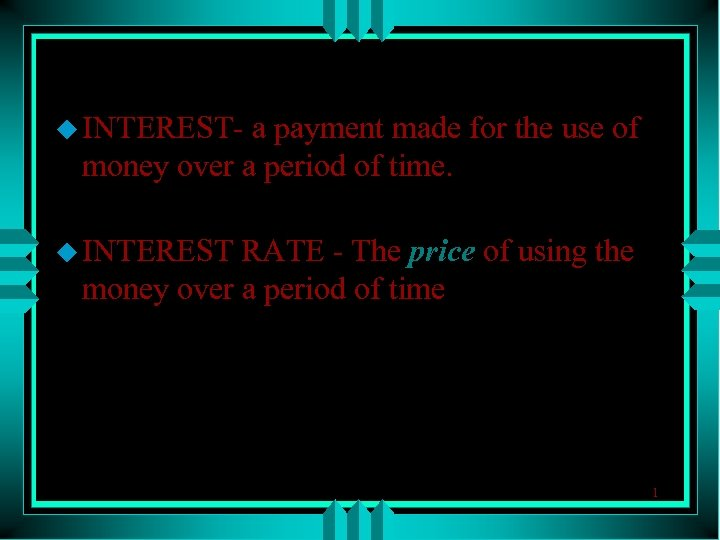u INTEREST- a payment made for the use of money over a period of time. u INTEREST RATE - The price of using the money over a period of time 1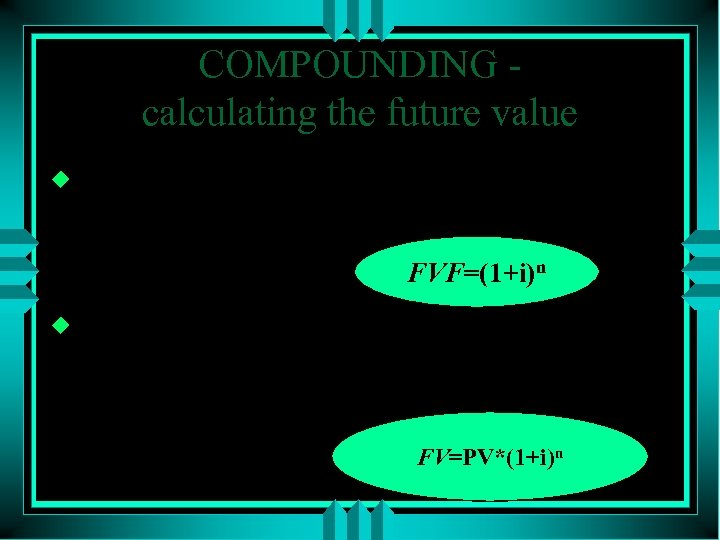COMPOUNDING calculating the future value u FUTURE VALUE FACTOR - The value by which a present value must be multiplied to calculate its future value FVF=(1+i)n u COMPOUNDING - Calculation of the future value of a present sum accounting for the rate of interest FV=PV*(1+i)n 2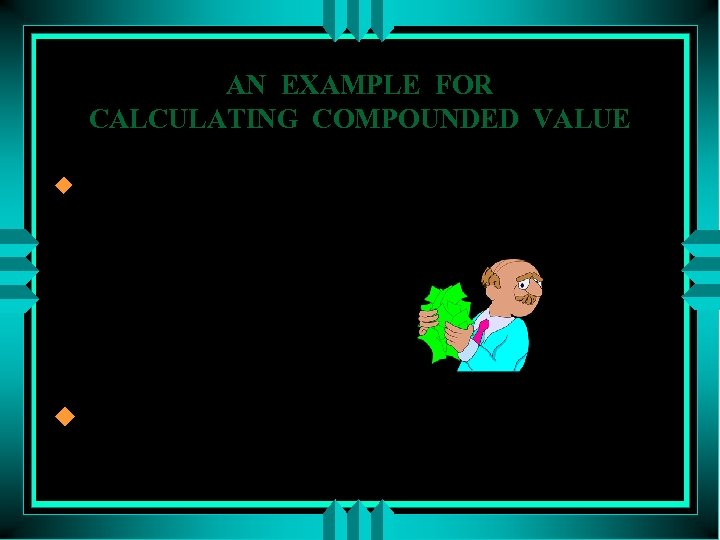AN EXAMPLE FOR CALCULATING COMPOUNDED VALUE u What is the future value of 4, 000\$ after 8 months, where the compound interest rate is 2% per month pv=4, 000 i=0. 02 n=8 fv=? u F. V. F=(1+0. 02)8=1. 1717 FV=4, 000*1. 1717=4, 686. 6 3DISCOUNTING – calculating the present value u Present Value Factor - The value by which a future value must be multiplied to calculate its present value PVF= u Discounting - Calculation of 1 (1+i)n the present value of the future sum 4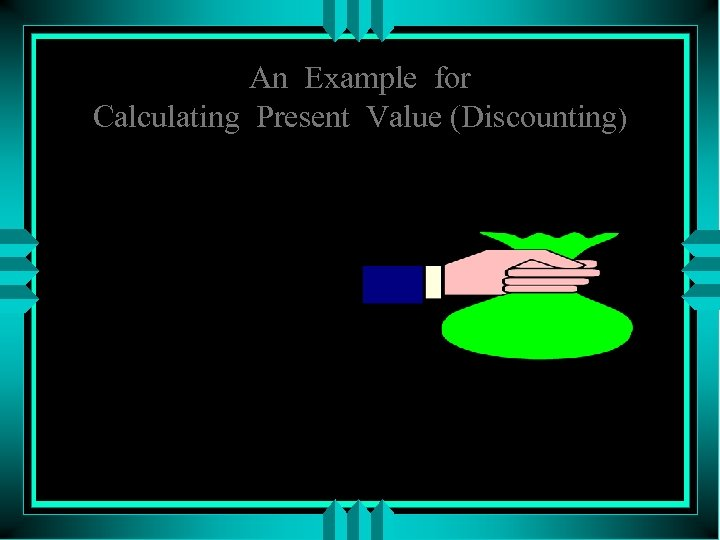An Example for Calculating Present Value (Discounting) What is the present value of \$2000 due in 10 years at 5% ? fv=2000 i=0. 05 n=10 pv=? pvf=1/ (1+0. 05)10 =0. 6139 pv=2000*0. 6139 =1227. 83 5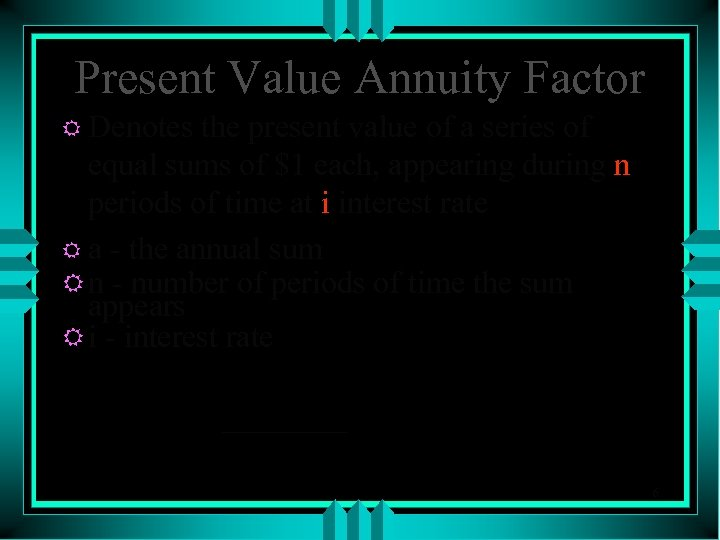Present Value Annuity Factor R Denotes the present value of a series of equal sums of \$1 each, appearing during n periods of time at i interest rate R a - the annual sum R n - number of periods of time the sum appears R i - interest rate pvaf= (1+i)n-1 i*(1+i)n 6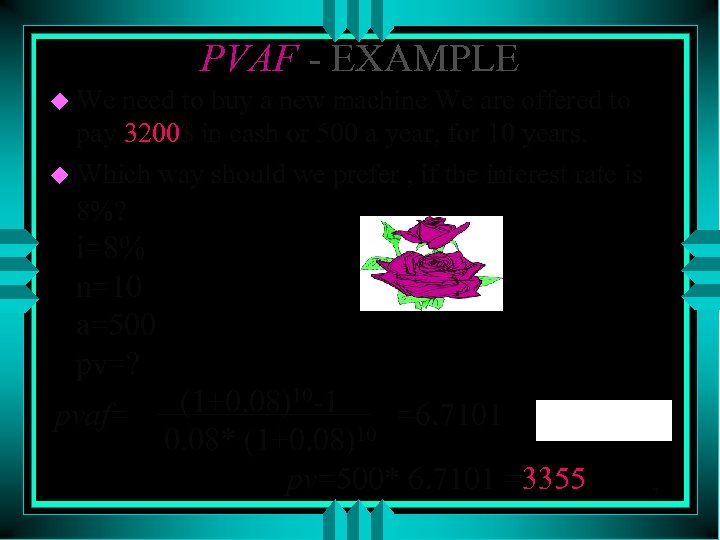PVAF - EXAMPLE u We need to buy a new machine We are offered to pay 3200\$ in cash or 500 a year, for 10 years. u Which way should we prefer , if the interest rate is 8%? i=8% n=10 a=500 pv=? pvaf= (1+0. 08)10 -1 =6. 7101 0. 08* (1+0. 08)10 pv=500* 6. 7101 =3355 7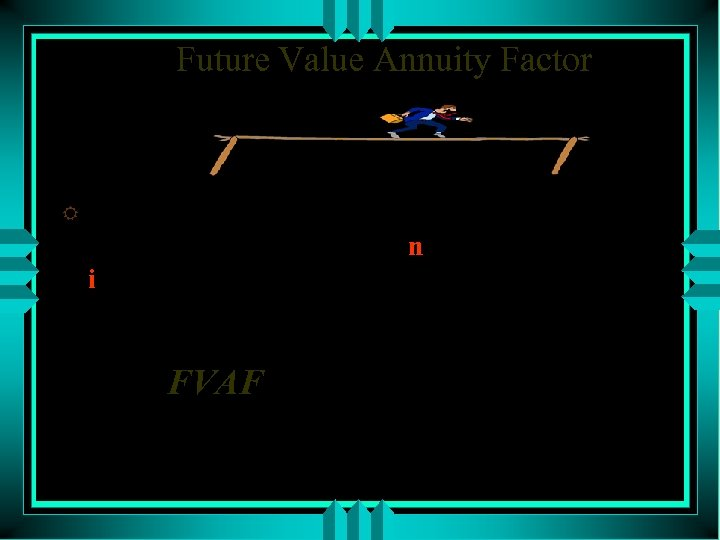Future Value Annuity Factor R Denotes the future value of a series of sums of \$1 each, appearing during n periods of time at i interest rate. FVAF= (1+i)n-1 i 8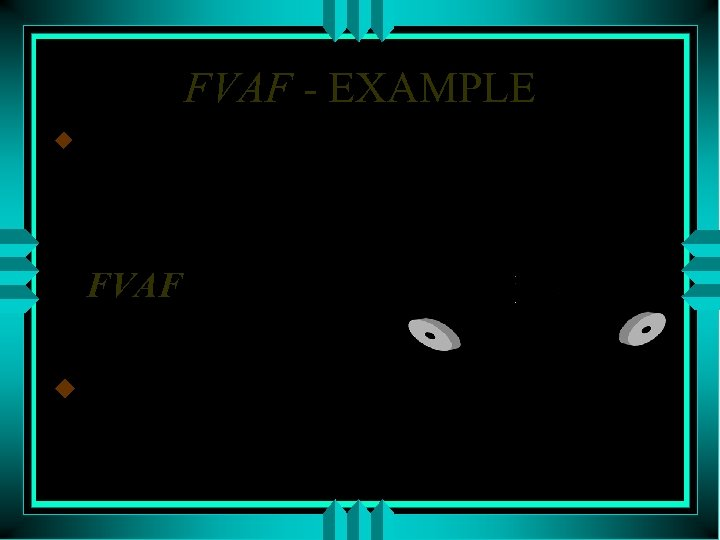FVAF - EXAMPLE u We want to save money to buy a new asset in 8 years. The asset’s price is 3, 000\$ interest rate is 5%. Is 300\$ a year enough? FVAF(5%, 8)=9. 5491 300*9. 5491=2864. 73 u Conclusion - 300\$ a year would not be enough. 9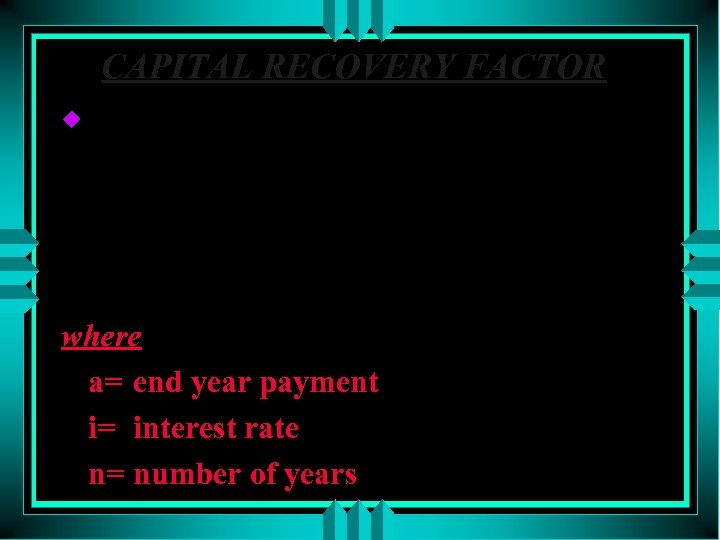CAPITAL RECOVERY FACTOR u is used to distribute a single amount invested today over a uniform series of end year payments which have a present value equal to the amount invested today. i*(1+i) n CRF= (1+i) n - 1 where a= end year payment i= interest rate n= number of years 10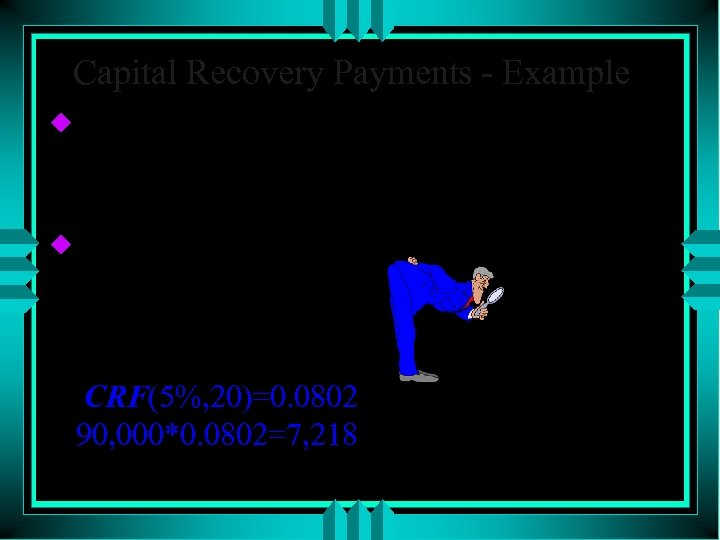Capital Recovery Payments - Example u. A loan of 90, 000\$ was taken for 20 years at an interest rate of 5% a year, what are the annual payment required to recover the loan. u pv=90, 000 n=20 i=5% a=? CRF(5%, 20)=0. 0802 90, 000*0. 0802=7, 218 11